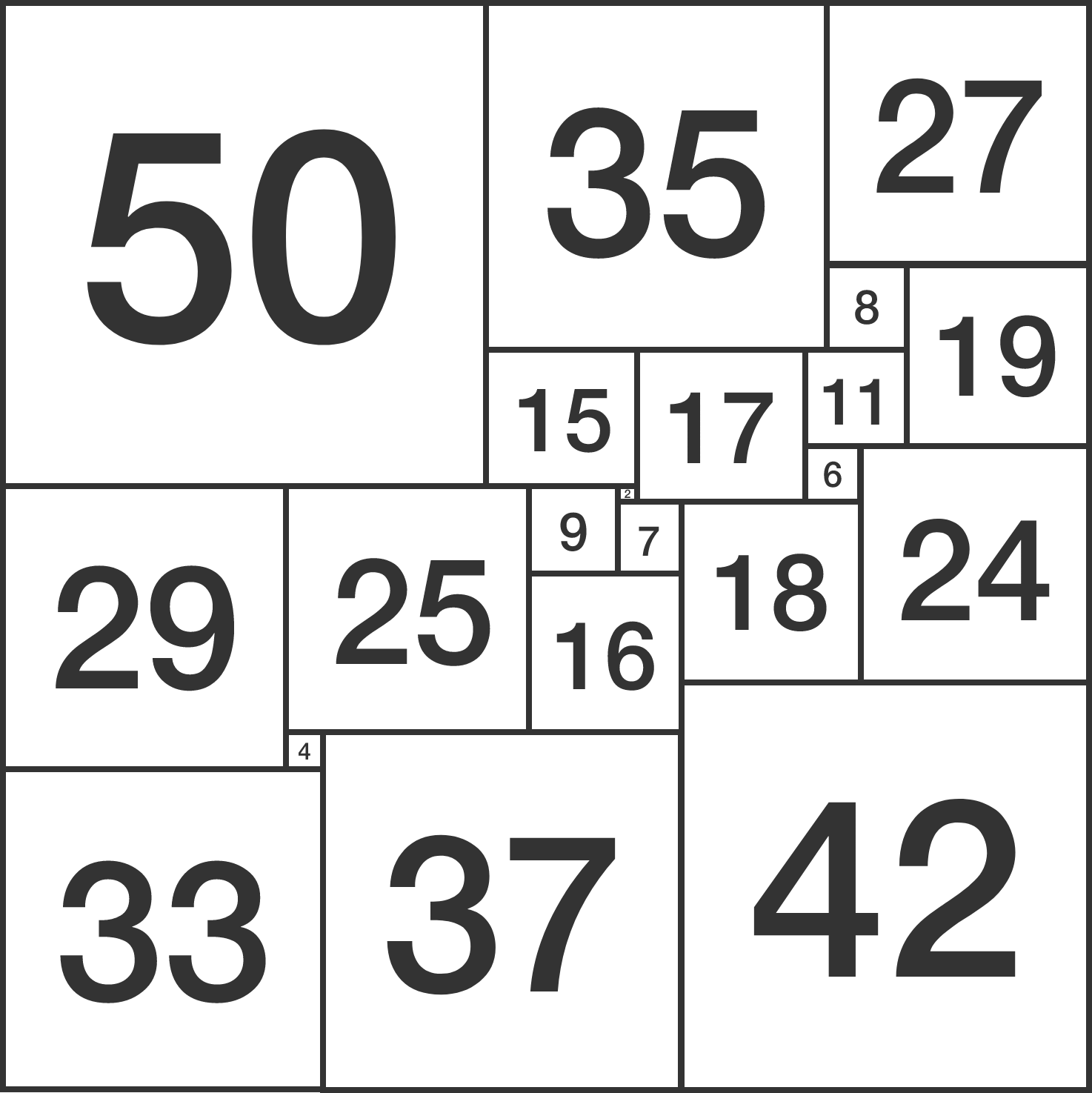# One Dimension Up

Probability Level 4As shown above, a square can be dissected into squares of distinct integer side lengths.

Is it possible to dissect a cube into cubes of distinct integer side lengths?


Clarification: The number in each square is the side length of that square.

×